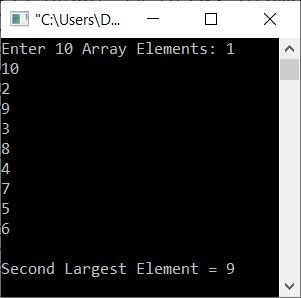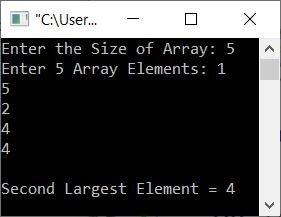# C++ Program to Find the Second Largest Element in an Array

This article provides programs in C++ to find and print the second largest element of an array entered by the user. The program is created using both for and while loops.

## Find the second largest element using the for loop

The question is, "Write a C++ program to find and print the second largest element in a given array." The program given below is its answer:

```#include<iostream>

using namespace std;
int main()
{
int i, arr, large, slarge;
cout<<"Enter 10 Array Elements: ";
for(i=0; i<10; i++)
cin>>arr[i];
large = arr;
for(i=0; i<10; i++)
{
if(large<arr[i])
large = arr[i];
}
slarge = arr;
for(i=0; i<10; i++)
{
if(slarge<arr[i])
{
if(arr[i]!=large)
slarge = arr[i];
}
}
cout<<"\nSecond Largest Element = "<<slarge;
cout<<endl;
return 0;
}```

The snapshot given below shows the initial output produced by the above C++ program on finding and printing the second largest element from the given array:Now supply the input, say 1, 10, 2, 9, 3, 8, 4, 7, 5, and 6 as ten elements, and press the ENTER key to find and print the second largest element from the given array, as shown in the snapshot given below:In the above program,

• I've assumed the very first element is the largest one.
• That is, the first element at the 0th index gets initialized to large.
• Now, using the value "large," I've checked with each and every element of the array.
• While checking, if the value of large is found to be less than any element, then I've initialized that element as the new value of large.
• In this way, the largest element gets stored in large.
• Now again, I've supposed that the very first element is the second-largest element. That is, "slarge" has the highest value this time.
• Now again, I've done the same job of comparing the value of slarge with each and every element of the array.
• But this time, if the value in slarge is found to be lesser, then the element gets initialized as the new value of slarge only when the element is not equal to large.
• In this way, the second-largest element gets found out and stored in slarge variable.
• Therefore, after exiting from the loop, just print the value of slarge as the second largest element of the array.

Now the question is, what if the user doesn't want to enter 10 elements?
That is, what if the user wants to enter some n elements, and based on those n elements, he/she wants to find the second largest element?
So we've got to modify the program.

Therefore, let's modify the above program and create another program that allows the user to define the size of the array along with its elements of a given size, as shown in the following program:

```#include<iostream>

using namespace std;
int main()
{
int tot, i, arr, large, slarge;
cout<<"Enter the Size of Array: ";
cin>>tot;
cout<<"Enter "<<tot<<" Array Elements: ";
for(i=0; i<tot; i++)
cin>>arr[i];
large = arr;
for(i=0; i<tot; i++)
{
if(large<arr[i])
large = arr[i];
}
slarge = arr;
for(i=0; i<tot; i++)
{
if(slarge<arr[i])
{
if(arr[i]!=large)
slarge = arr[i];
}
}
cout<<"\nSecond Largest Element = "<<slarge;
cout<<endl;
return 0;
}```

Here is its sample run with user input of 5 as size and 1, 5, 2, 4, 4 as five elements:## Find the second largest element using the while loop

Except for the approach, this program is nearly identical to the previous one. This program is created using the while loop. The rest of the things are exactly the same as in the previous program.

```#include<iostream>

using namespace std;
int main()
{
int tot, i=0, arr, large, slarge;
cout<<"Enter the Size of Array: ";
cin>>tot;
cout<<"Enter "<<tot<<" Array Elements: ";
while(i<tot)
{
cin>>arr[i];
i++;
}
large = arr;
i=0;
while(i<tot)
{
if(large<arr[i])
large = arr[i];
i++;
}
slarge = arr;
i=0;
while(i<tot)
{
if(slarge<arr[i])
{
if(arr[i]!=large)
slarge = arr[i];
}
i++;
}
cout<<"\nSecond Largest Element = "<<slarge;
cout<<endl;
return 0;
}```

This program does exactly the same job as the previous program.

C++ Quiz

« Previous Program Next Program »